# 斐波那契数列

+关注继续查看

C++

 1 class Solution {
2 public:
3     int Fibonacci(int n) {
4         if (n == 0) return 0;
5         if (n <= 2) return 1;
6
7         int a = 1, b = 1;
8         while(n-- > 2) {
9             a = a + b;
10             b = a - b;
11         }
12         return a;
13
14     }
15 };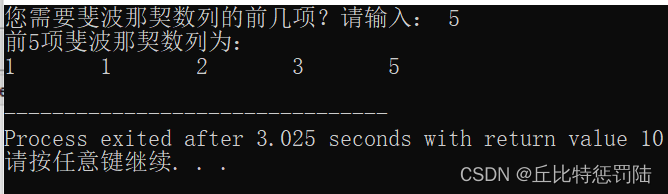29 024 031 0Fibonacci斐波那契数列的几种题型
Fibonacci斐波那契数列的几种题型
21 0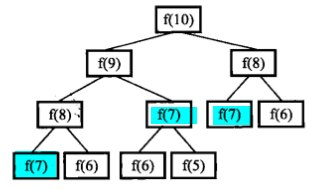30.斐波那契数列

21 0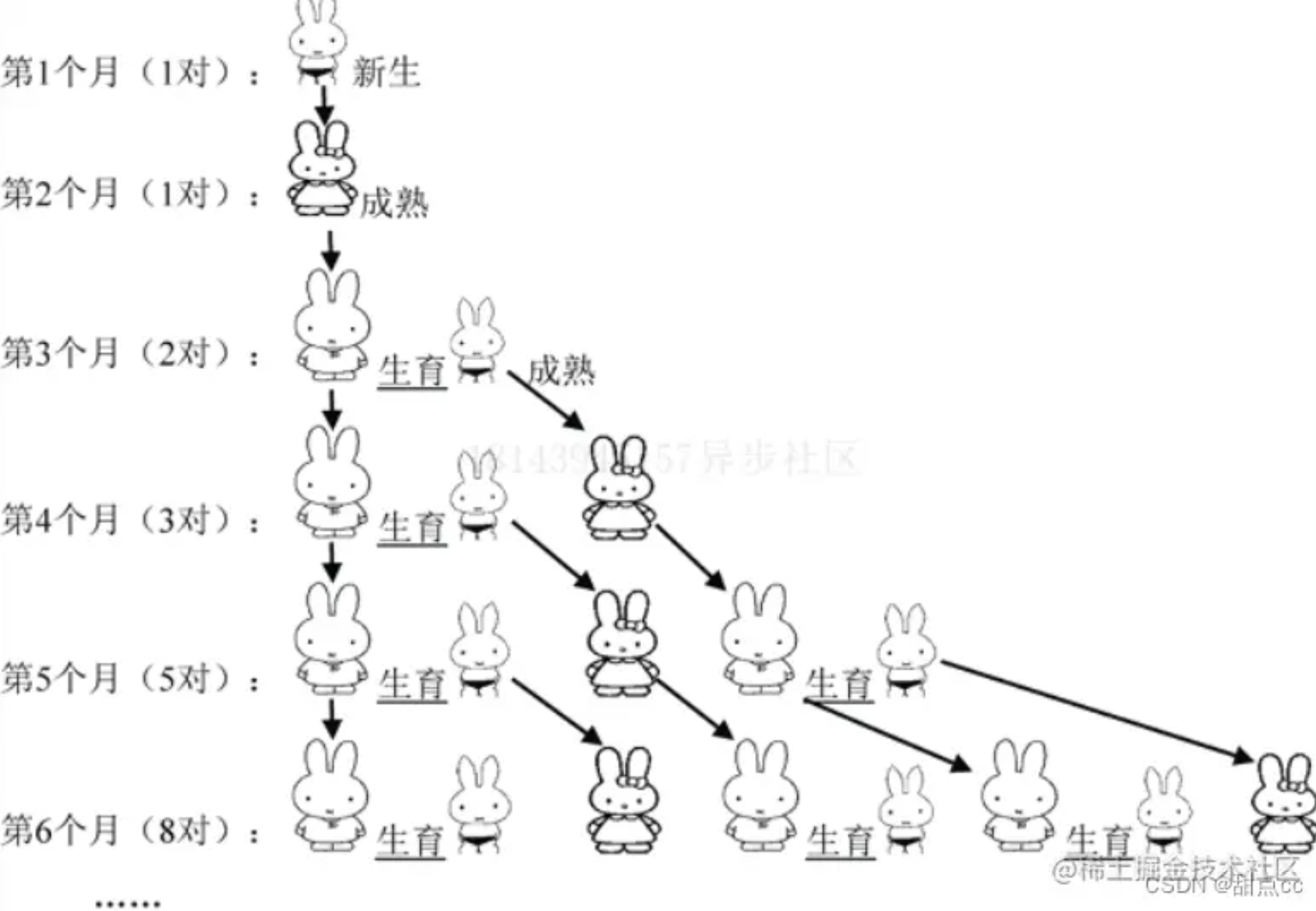26 0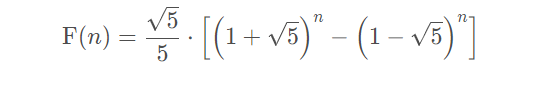33 0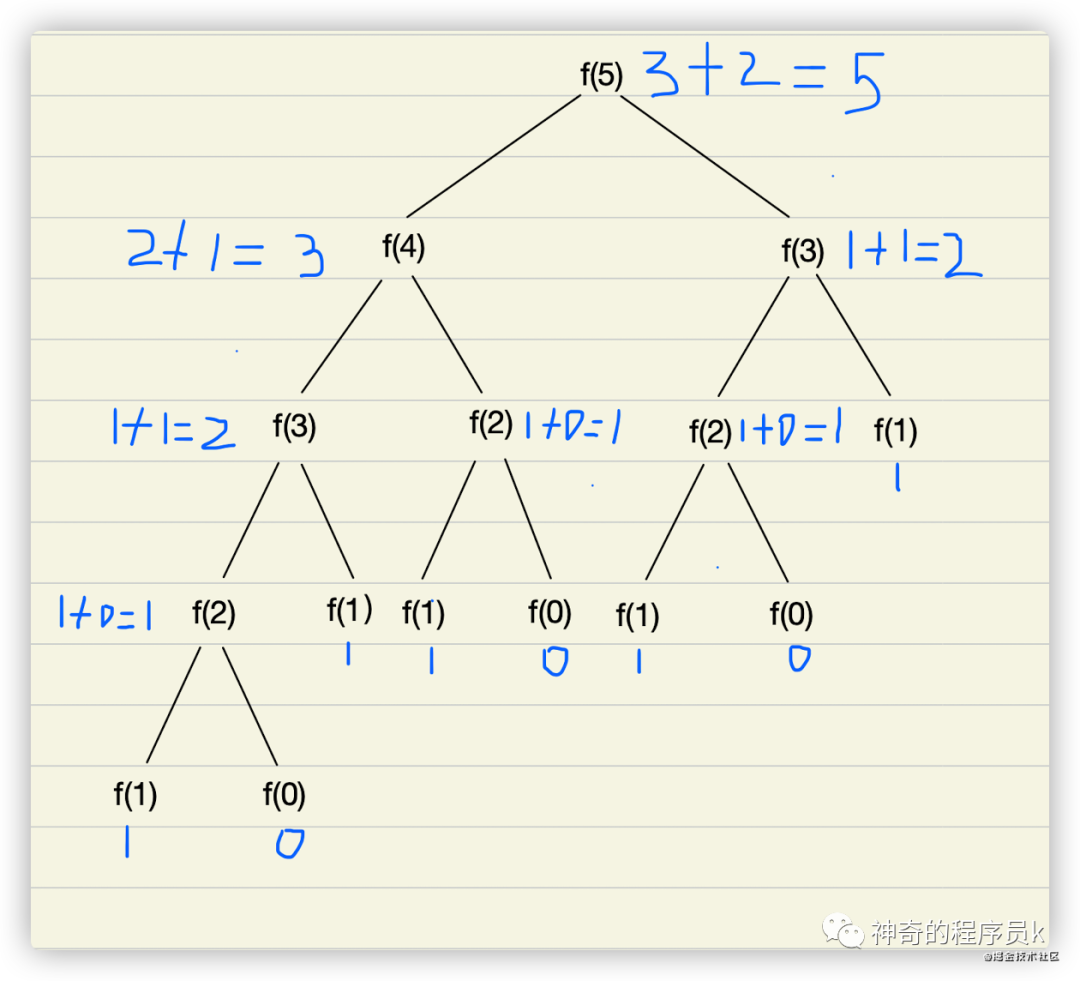41 0